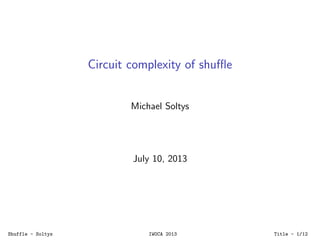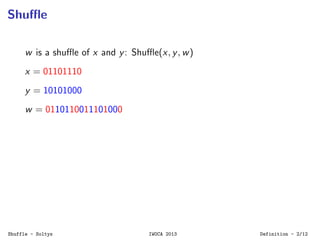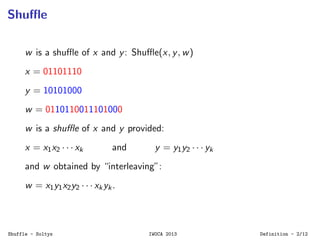Successfully reported this slideshow.

# Circuit Complexity of Shuffle - IWOCA 2013×

1 of 13
1 of 13

# Circuit Complexity of Shuffle - IWOCA 2013

Presentation at the International Workshop on Combinatorial Algorithms, IWOCA 2013.

Presentation at the International Workshop on Combinatorial Algorithms, IWOCA 2013.

## More Related Content

### Circuit Complexity of Shuffle - IWOCA 2013

1. 1. Circuit complexity of shuﬄe Michael Soltys July 10, 2013 Shuffle - Soltys IWOCA 2013 Title - 1/12
2. 2. Shuﬄe w is a shuﬄe of x and y: Shuﬄe(x, y, w) x = 01101110 y = 10101000 w = 0110110011101000 Shuffle - Soltys IWOCA 2013 Definition - 2/12
3. 3. Shuﬄe w is a shuﬄe of x and y: Shuﬄe(x, y, w) x = 01101110 y = 10101000 w = 0110110011101000 w is a shuﬄe of x and y provided: x = x1x2 · · · xk and y = y1y2 · · · yk and w obtained by “interleaving”: w = x1y1x2y2 · · · xkyk. Shuffle - Soltys IWOCA 2013 Definition - 2/12
4. 4. Motivation Modelling and veriﬁcation of concurrent systems Used in XML database systems for schema deﬁnitions Plan recognition Natural language processing See http://bit.ly/17OD2md for more details Shuffle - Soltys IWOCA 2013 Motivation - 3/12
5. 5. Square Shuﬄe w is a square provided it is equal to a shuﬄe of a x with itself, i.e., ∃x s.t. Shuﬄe(x, x, w). The string w = 0110110011101000 is a square: w = 0110110011101000 and x = 01101100 = 01101100 Shuffle - Soltys IWOCA 2013 Square Shuffle - 4/12
6. 6. given an alphabet Σ, |Σ| ≥ 7, Square = {w : ∃x Shuﬄe(x, x, w)} is NP-complete; see http://arxiv.org/abs/1211.7161 What we leave open: What about |Σ| = 2 (for |Σ| = 1, Square is just the set of even length strings) What about if |Σ| = ∞ but each symbol cannot occur more often than, say, 6 times (if each symbol occurs at most 4 times, Square can be reduced to 2-Sat – see P. Austrin Stack Exchange post http://bit.ly/WATco3) Shuffle - Soltys IWOCA 2013 Square Shuffle - 5/12
7. 7. Upper Bound Shuﬄe(000, 111, 010101) Shuﬄe(011, 011, 001111) Based on Mansﬁeld’s algorithm, Shuﬄe ∈ NL Shuffle - Soltys IWOCA 2013 Upper bound - 6/12
8. 8. Circuits 5.1. BASIC RESULTS AND DEFINITIONS 67 ¬ OO _ OO ^ OO ^ OO GG ¬ ?? ¬ __ >> `` OO 66 OO KK Figure 1. Using de Morgan and replicating gates. _ OO _ OO ?? __GG WWShuffle - Soltys IWOCA 2013 Upper bound - 7/12
9. 9. Suppose that we want a family of circuits that recognize {1n : n ∈ N} ⊆ {0, 1}∗. 4x x x x x x x x x x1 21 1 12 23 3 I.e., it can be done with a ciruit family {Ci } where |Ci | ≤ 1 (and depth 1), and hence in AC0 . Shuffle - Soltys IWOCA 2013 Upper bound - 8/12
10. 10. By results of Sudborough & Venkateswaran: NL ⊆ SAC1 ⊆ AC1 Shuffle - Soltys IWOCA 2013 Upper bound - 9/12
11. 11. Lower Bound #(x)s be the number of occurrences of a symbol s in the string x. Shuﬄe(0#(x)0 , 1#(x)1 , x) is always true. Parity(x) = 0 ≤ i ≤ |x| i is odd Shuﬄe(0|x|−i , 1i , x), Shuffle - Soltys IWOCA 2013 Lower bound - 10/12
12. 12. n−i i=1 i=3 i=5 i=n 0 x 1 1 10 0 0x x x1 ii n−i i in−i n−i Shuffle - Soltys IWOCA 2013 Lower bound - 11/12
13. 13. By the famous result of Furst, Saxe, Sipser, Parity ∈ AC0 . Therefore Shuﬄe ∈ AC0 . Shuffle - Soltys IWOCA 2013 Lower bound - 12/12